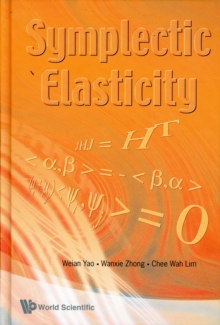Supporting your high street Find out how »
• My AccountSymplectic Elasticity Hardback

Description

Exact analytical solutions in some areas of solid mechanics, in particular problems in the theory of plates, have long been regarded as bottlenecks in the development of elasticity.

In contrast to the traditional solution methodologies, such as Timoshenko's approach in the theory of elasticity for which the main technique is the semi-inverse method, this book presents a new approach based on the Hamiltonian principle and the symplectic duality system where solutions are derived in a rational manner in the symplectic space.

Dissimilar to the conventional Euclidean space with one kind of variables, the symplectic space with dual variables thus provides a fundamental breakthrough.

A unique feature of this symplectic approach is the classical bending problems in solid mechanics now become eigenvalue problems and the symplectic bending deflection solutions are constituted by expansion of eigenvectors.

The classical solutions are subsets of the more general symplectic solutions.This book explains the new solution methodology by discussing plane isotropic elasticity, multiple layered plate, anisotropic elasticity, sectorial plate and thin plate bending problems in detail.

A number of existing problems without analytical solutions within the framework of classical approaches are solved analytically using this symplectic approach.

Symplectic methodologies can be applied not only to problems in elasticity, but also to other solid mechanics problems.

In addition, it can also be extended to various engineering mechanics and mathematical physics fields, such as vibration, wave propagation, control theory, electromagnetism and quantum mechanics.

Information

• Format: Hardback
• Pages: 316 pages
• Publisher: World Scientific Publishing Co Pte Ltd
• Publication Date:
• Category: Classical mechanics
• ISBN: 9789812778703

£97.00

£78.69

on all orders

Pick up orders

from local bookshops